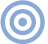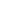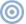Features

Quality Lies in the Details SIDEBAR 1: Decibels

SIDEBAR 1: Decibels
The term decibel (dB) appears repeatedly in specifications and measurements, yet it's often misunderstood. In addition, there seem to be all kinds of dB: dBm, dBR, dBV, dBFS, dBW, and dB SPL, to name a few. What do they all mean?

The most important thing to realize is that the decibel doesn't express an absolute value—it expresses a ratio between two values. When we say that a particular CD player has a 2.5dB higher output voltage than another CD player, we're expressing the difference in output voltages between the players. In the case of our CD players, it's the ratio of their respective output voltages that's expressed by the decibel amount. Any time the term decibel is used, it must be accompanied by a reference level—either to another level, as with the two CD players, or against a standard reference.

This is where all those confusing suffixes come in. The letters after the number of dB (+4dBm or -90dBFS, for example) denote the reference level to which the expressed value is referenced. For example, when we plot a digital processor's output spectrally when decoding a -90dBFS, 1kHz sinewave, the letters "FS" tell us that the test-signal voltage is 90dB below "Full Scale" digital (the maximum level). 90dB is the difference between full-scale amplitude and the test-signal amplitude. Although we don't always include the suffix, it's assumed from the context. When expressing digital signal levels, it's commonly known that the reference level is full-scale, or 0dBFS.

The expression "dBm," found mostly in professional equipment measurements and specifications, means that the reference level is 0.775 volts. The "m" in "dBm" comes from the word "milliwatt": 1mW of power is dissipated when 0.775V is applied across 600 ohms (a standard impedance). If a tape recorder puts out +4dBm at line level (0VU), we know that its output voltage is 4dB above 0.775V (which, incidentally, is 1.228V).

If we say a certain sound-pressure level (spl) is 94dB spl, the suffix "spl" tells us that the sound pressure is referenced to 0dB spl—the threshold of hearing. When you see a loudspeaker-sensitivity measurement that indicates that the loudspeaker measured at 1 meter away will produce 89dB spl for an input voltage of 2.828V, we know that the loudspeaker's output is 89dB above the reference level of 0dB spl. Incidentally, 0dB spl is a pressure on your eardrum of 0.0002 dynes/cm^2, which is the threshold of audibility. This value is so minuscule that it's sometimes humorously referred to as "the weight of a gnat's eyelash."

The point is that any expression in decibels is always a relationship between two values, whether they're two voltages, two electrical powers, or two sound-pressure levels.

Calculating decibel differences is easy. All you need is a calculator that will find the logarithm (to the base 10) of a number. Here's how to find the number of dB difference in output level between two components: Let's say you're matching levels between digital processors before auditioning them (a very wise practice) and want to make sure they're matched to within, say, 0.2dB. The first processor produces an output voltage from the preamp (with its volume control at a comfortable listening level) of 85mV when reproducing a -20dBFS, 1kHz sinewave (track 1 of the first Stereophile Test CD). The second processor produces 91mV at the preamplifier output with the same volume setting. How much difference is there in dB between the two processors? Are they close enough to meet the 0.2dB matching requirement, or does the preamp need turning down for the second processor?

First, find the ratio between the two voltages by dividing 91 by 85. This tells us that the ratio between the two voltages is 1.071:1. Next, find the log of 1.071 by pressing the "log" button on the calculator with 1.071 in the display. You should get 0.0296—the log of 1.071. Now multiply the log of the two voltages (0.0296) by 20. The answer, 0.592, is the difference in decibels between those two voltages. The two processors are matched to within just under six-tenths of a dB (which is not enough). Expressing this equation verbally, it's "Number of dB equals twenty times the log (to the base ten) of the ratio between the two voltages" (footnote 1).

Calculating power differences is slightly different. Instead of multiplying the log of the ratio of the two values by 20, we multiply by 10. Let's say one power amplifier has a maximum output at clipping of 134W, and another has a maximum output power of 268W. What's the decibel difference between them? First find the ratio between the two powers by dividing 268 by 134. The answer is 2. Find the log of 2 (0.301) and multiply by 10. The answer is that the second amplifier has 3dB more output power than the first amplifier. Note that a doubling of power yields an increase of 3dB, and a doubling of voltage represents an increase of 6dB. By extrapolation, we can see that four times the voltage represents an increase of 12dB, eight times the voltage is 18dB, 16 times the voltage is 24dB, and so on in a logarithmic progression.

Why do we use the decibel notation to express electrical powers, voltages, and sound-pressure levels? There are two very good reasons. First, the logarithmic nature of decibel notation corresponds very closely with human perception. An increase in spl from 60dB to 63dB is subjectively the same as an increase from 100dB to 103dB, even though the latter increase represents a vastly greater linear change in pressure. This is analogous to the way we perceive pitch: we hear each doubling of frequency as an octave, yet each ascending octave spans a frequency difference twice that of the next-lower octave. The octave span between 20Hz and 40Hz is perceived as the same interval as the octave between 10kHz and 20kHz, although the latter is a much larger change in frequency.

Second, decibel notation makes expressing huge numbers more convenient. It is far easier to say that the threshold of pain is 130dB spl than to say that the threshold of pain is 10,000,000,000,000 (10^13) times as loud as the threshold of hearing (footnote 2).

The decibel makes working with audio easier. Not only does the dB notation correspond nicely to our perception of sound, it also makes expressing and calculating large numbers more convenient.—Robert Harley

Footnote 1: For voltages, NdB = 20 log V1/V2.

Footnote 2: It is truly awe-inspiring to realize that our ears' dynamic range (threshold of hearing to threshold of pain) spans a level difference of 10 trillion times.ARTICLE CONTENTSX